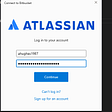# Difference Between Var, Let and Const in ES6

## The Difference with Handy examples

The differences between these three variables made me confused when I started to use ECMAScript 6. The most confusing was to understand the difference between let and var.

So let’s try to explain this difference.

`var a = 'I am var';const b = 'I am const';let c = 'I am let';`

# What are the differences?

At first, let’s explain the concept of scope.

The scope is the fundamental concept in all programming languages ​​that defines the visibility of a variable.
The variables can be globals or locals.

# How var is working

var defines a variable globally regardless of the block scope.

Let’s see some practical example with the if condition and the for loop

## if

`var exampleVar = "out";if (true) {    var exampleVar = "in";    console.log(exampleVar);}console.log(exampleVar);`

Here the output is:

`inin`

That’s because of the var inside the if block that override the first variable.

## for

`var exampleForVar = 1;for (var exampleForVar = 0; exampleForVar < 5; exampleForVar++) {     console.log('in: ',exampleForVar);}console.log('out: ',exampleForVar);`

Here the output is:

`in: 0in: 1in: 2in: 3in: 4out: 5`

This because the declaration inside the loop replaces the one outside.

Probably this example doesn’t tell you anything but if you will look the same examples with the variable let it will be obvious.

# How let is working

let allows you to declare variables that are limited in scope to the block, statement of expression.

Let’s see some practical example with the if condition and the for loop

## if

`let exampleLet = "out"; if (true) {    let exampleLet = "in";     console.log(exampleLet);}console.log(exampleLet);`

Here the output is:

`inout`

Here you can notice the difference, above with var we had as output “in” “in” but now is “in” “out”. That’s because the let variable keeps the declaration inside the scope.

for

`let exampleForLet = 1;for (let exampleForLet = 0; exampleForLet < 5; exampleForLet++) {     console.log('in: ',exampleForLet);}console.log('out: ',exampleForLet);`

Here the output is:

`in: 0in: 1in: 2in: 3in: 4out: 1`

Also here we can notice the difference, it’s clear the separation between the variable outside and the one inside the block.

But they are working in the same way when we use a function:

`var myVar = "out var";let myLet = "out let";function readVariable() {    var myVar = "in var";    let myLet = "in let";    console.log(myVar);        console.log(myLet);}readVariable();console.log(myVar);console.log(myLet);`

Here the output is:

`in varin letout varout let`

As you can see in this case, the var is not overriding the variable outside of the function as the previous example.

# How const is working

Now let’s see the most simple of these variables const.

It is an immutable variable, so you can’t change it anymore once it is declared within the same scope, we can see it with an example

`const exampleConst = “first declaration”;exampleConst = “Second declaration”;`

This code return an error:

`SyntaxError: Identifier ‘exampleConst’ has already been declared`

but if we do the same with let and var, we wouldn’t get this error, that’s because var and let allow you to change their values depending on their values.

It’s different if we are dealing with an array or an object.

Array

`const arrayVariables = ['var', 'let']; arrayVariables.push('const'); console.log(arrayVariables); `

output:

`['var', 'let', 'const']`

Object

`const objectConst = { keyword: 'var' }; objectConst.keyword = 'const';console.log(objectConst); `

output:

`{ keyword: 'const' }`

As we can see, only when our const is an array or an object we can change its value.

# Recap

• Var: defines a variable globally regardless of the block scope.
• Let: let allows you to declare variables that are limited in scope to the block, statement of expression.
• Const: it’s an immutable variable where you can’t change its value once declared.

I hope these examples helped you to understand the difference better if you have feedback or question about this topic I would be happy to answer 😃

## More from NotOnlyCSS

This publication includes original articles and tips about frontend technologies.## Solazzo Nicola

Full-stack developer Javascript for Starteed.com

## How TomTom Maps APIs Enable Just-in-Time Construction Material Delivery## Local Development in Bitbucket With App Password## How to push your code to GitHub using few git commands## In 2022, learn the 7 best Scala frameworks and libraries for concurrency, web development, and big…